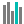#LOGEST (PQL - xl)

This function calculates an exponential curve that fits the data and returns an array of values that describes the curve

• Library: PQL \ Spreadsheet \ Statistical
• Compatibility: Any content (regardless of data source) in the Tabulate spreadsheet module

#### Syntax

LOGEST(known ys, OPTIONAL known xs, const, stats)

##### Function Arguments
 Name Description Type Optional known ys Set of y values that are known in the relationship y=b*m^x StringOrNumber known xs X is an optional set of x values that may be known in the relationship y=mx+b StringOrNumber Y const Logical value that specifies whether to force the constant b to equal 0. If true or omitted then b is calculated normally; if false then b is equal to 0 and the m values are adjusted so that y=m^x StringOrNumber stats Logical value that specifies whether to return additional regression statistics. If true, then the additional regression statistics are returned if false or omitted then only the m-coefficients and b are returned StringOrNumber